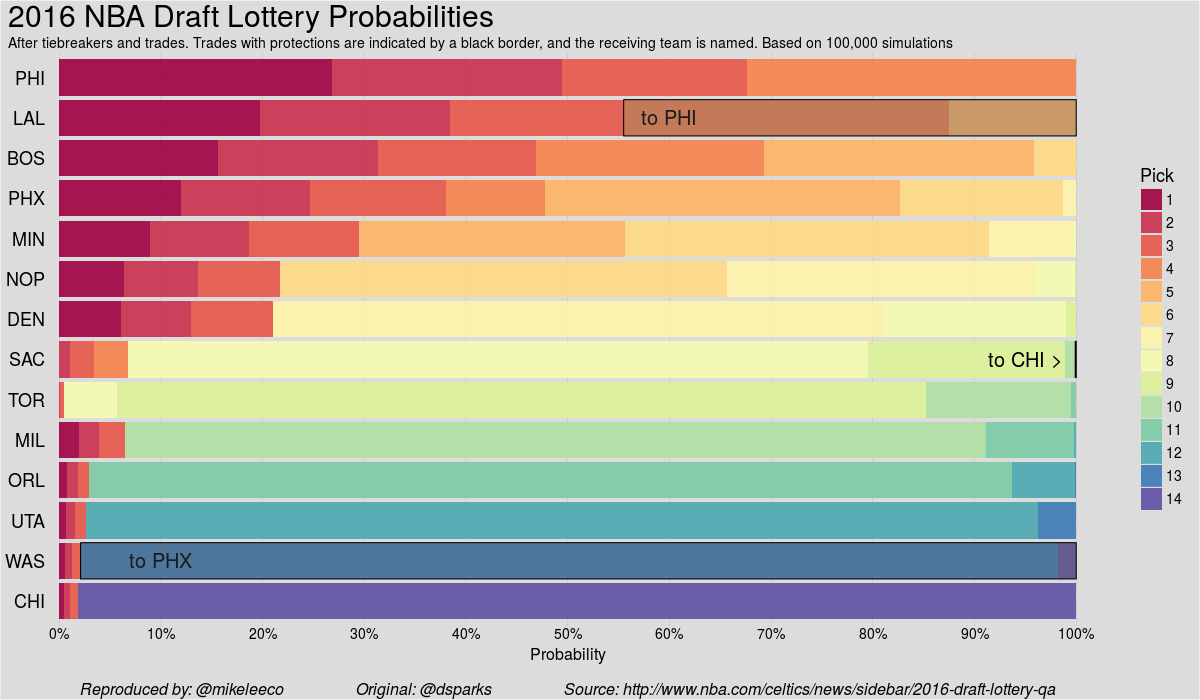# NBA Draft Odds Plot

#### June 1, 2016

Original Source

I was scrolling twitter and noticed this tweet from David Sparks - Director of Basketball Analytics for the Boston Celtics:

With his obligatory shoutouts to Hadley Wickham and BoB Rudis, I felt compelled to reproduce the plot in RStudio and get some ggplot2 code out there open-sourcing his work.

Package Dependencies and Data

The data was generated using simulations of the NBA Draft lottery, which resulted in some peculiar distributions compared to the raw lottery odds. I fudged some of the data based on how it looked in the plot - not much I can do about that since there wasn’t anything programmatically to work from there! `¯\_(ツ)_/¯`

``````#devtools::install_github("hadley/ggplot2")
library(ggplot2)
library(tidyr)
library(RCurl)
library(RColorBrewer)
dataurl <- 'https://gist.githubusercontent.com/mikeleeco/268429d0b4019ec30c4e547c16d7f766/raw/afa955af265d14a2f72780e4e0b87ffe469c0109/odds.csv'
data <- getURL(dataurl)
``````

Data Cleanup

The tidyr package allows us to reshape our data between long and wide formats, RColorBrewer helps generate a fluid color pallete for our plot, and RCurl assists in sourcing the data from my gist.

``````odds <- gather(odds, X)
odds[,2] <- substring(odds[,2], 2)
colnames(odds) <- c("Team","Pick","Probability")
odds\$Pick <- as.numeric(odds\$Pick)
odds\$Team <- as.character(odds\$Team)
odds\$Team <- factor(odds\$Team, levels = odds[1:14,1])
odds\$Team <- factor(odds\$Team, levels=rev(levels(odds\$Team)))
odds\$Pick <- factor(odds\$Pick, levels = 1:14)
getPalette <- colorRampPalette(brewer.pal(11, "Spectral"))(14)
odds\$Probability <- ifelse(is.na(odds\$Probability),0,odds\$Probability)
``````

The `colorRampPallete` and `brewer.pal` functions expands the “Spectral” color pallete from 11 to 14 colors, and we’ve organized our Team y-axis to be leveled in order of likelihood of winning the top pick - without factoring in the projections that were used in the @celtics figure.

The Custom Plot Theme

Now that we’ve prepared our data, we can get to customizing the plot!

``````g <- ggplot(odds, aes(Team))
g <- g + geom_bar(aes(x = Team, y = Probability, fill = Pick),alpha=0.9,stat="identity")
g <- g + scale_y_continuous(breaks = seq(0, 1, by = 0.1),1,name="Probability", labels=scales::percent) +
labs(x=NULL, y=NULL, title="2016 NBA Draft Lottery Probabilities",
subtitle = "After tiebreakers and trades. Trades with protections are indicated by a black border, and the receiving team is named. Based on 100,000 simulations",
caption="Reproduced by: @mikeleeco                  Original: @dsparks                  Source: http://www.nba.com/celtics/news/sidebar/2016-draft-lottery-qa") +
coord_flip() +
scale_fill_manual(values = getPalette)
``````

The development version of ggplot2 on github features numerous additions including ecpanded arguments for `labs` such as subtitles and captions. Check out `?ggplot2::labs` or Bob Rudis' post on his contribution to ggplot2.

``````g <- g  + theme(
axis.text.x = element_text(size=14,margin=margin(b=5),color = "black"),
axis.title.x = element_text(size=16),
plot.subtitle = element_text(size=14),
plot.caption = element_text(size=16,margin = margin(t=20),face = "italic", hjust = .5),
axis.text.y = element_text(size=18,margin = margin(r=-40),colour = "black"),
axis.ticks.y=element_blank(),
axis.ticks.x=element_blank(),
plot.title = element_text(size=30,margin = margin(b=10)),
panel.border=element_blank(),
panel.grid.major.x=element_line(color="#2b2b2b", linetype="dotted", size=0.15),
panel.grid.major.y=element_blank(),
legend.text = element_text(size=14),
legend.title = element_text(size=18),
legend.key = element_rect(fill="#DCDCDC",colour = "#DCDCDC"),
legend.background = element_rect(fill="#DCDCDC"),
panel.background = element_rect(fill="#DCDCDC"),
plot.background = element_rect(fill="#DCDCDC")
)
``````

As seen in the theme here, the developtmental version of ggplot2 also features elements to customize the new caption and subtitle arguments. Things are looking pretty sharp!

Annotations - Call for Help!

The lottery data has been transformed into a customized plot; the only missing pieces are the transparent rectangular grobs indicating picks with trade implications. I tried a few different methods to programmatically assign labels over the rows in our dataset, none of which gave the desired final output. Instead I used brute force to place transparent rectangles and texts over the appropriate data blocks:

``````g <- g + annotate("text", x = (13.45+12.55)/2, y = .6, alpha = 1,color="black", label="to PHI", size= rel(7)) +
annotate("rect", xmin = 12.55, xmax = 13.45, ymin = .555, ymax = 1, alpha = .3,color="black") +
annotate("text", x = (2.45+1.55)/2, y = .1, alpha = 1,color="black", label="to PHX", size= rel(7)) +
annotate("rect", xmin = 1.55, xmax = 2.45, ymin = .021, ymax = 1, alpha = .3,color="black") +
annotate("text", x = (7.45+6.55)/2, y = .95, alpha = 1,color="black", label="to CHI >", size= rel(7)) +
annotate("rect", xmin = 6.55, xmax = 7.45, ymin = .999, ymax = 1, alpha = .3,color="black")
``````

There has to be a more efficient method to do this! If you know of a better way please contact me on twitter or create a pull request on this post.

Final product: